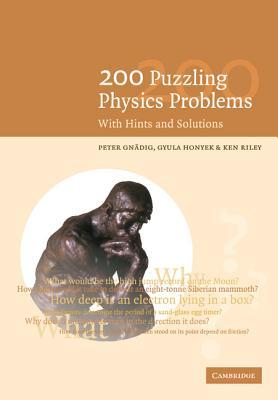# 匀速拉地毯最少需要多大的力？## 问题## 提示## 解答\begin{equation*} F=\frac{\mathrm dp}{\mathrm dt}=\frac{\mathrm dm}{\mathrm dt}v+\frac{\mathrm dv}{\mathrm dt}m=\frac{\mathrm dm}{\mathrm dt}1+0 \end{equation*}

### 添加新评论